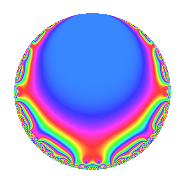Properties

 Label 4002.2.a.tLevel 4002 Weight 2 Character orbit 4002.a Self dual Yes Analytic conductor 31.956 Analytic rank 0 Dimension 2 CM No Inner twists 1

Related objects

Newspace parameters

 Level: $$N$$ = $$4002 = 2 \cdot 3 \cdot 23 \cdot 29$$ Weight: $$k$$ = $$2$$ Character orbit: $$[\chi]$$ = 4002.a (trivial)

Newform invariants

 Self dual: Yes Analytic conductor: $$31.9561308889$$ Analytic rank: $$0$$ Dimension: $$2$$ Coefficient field: $$\Q(\sqrt{2})$$ Coefficient ring: $$\Z[a_1, \ldots, a_{7}]$$ Coefficient ring index: $$1$$ Fricke sign: $$-1$$ Sato-Tate group: $\mathrm{SU}(2)$

$q$-expansion

Coefficients of the $$q$$-expansion are expressed in terms of $$\beta = \sqrt{2}$$. We also show the integral $$q$$-expansion of the trace form.

 $$f(q)$$ $$=$$ $$q$$ $$- q^{2}$$ $$- q^{3}$$ $$+ q^{4}$$ $$+ 2 \beta q^{5}$$ $$+ q^{6}$$ $$+ \beta q^{7}$$ $$- q^{8}$$ $$+ q^{9}$$ $$+O(q^{10})$$ $$q$$ $$- q^{2}$$ $$- q^{3}$$ $$+ q^{4}$$ $$+ 2 \beta q^{5}$$ $$+ q^{6}$$ $$+ \beta q^{7}$$ $$- q^{8}$$ $$+ q^{9}$$ $$-2 \beta q^{10}$$ $$+ 4 q^{11}$$ $$- q^{12}$$ $$+ ( 2 - 2 \beta ) q^{13}$$ $$-\beta q^{14}$$ $$-2 \beta q^{15}$$ $$+ q^{16}$$ $$-2 \beta q^{17}$$ $$- q^{18}$$ $$+ ( -4 - \beta ) q^{19}$$ $$+ 2 \beta q^{20}$$ $$-\beta q^{21}$$ $$-4 q^{22}$$ $$- q^{23}$$ $$+ q^{24}$$ $$+ 3 q^{25}$$ $$+ ( -2 + 2 \beta ) q^{26}$$ $$- q^{27}$$ $$+ \beta q^{28}$$ $$+ q^{29}$$ $$+ 2 \beta q^{30}$$ $$+ 6 q^{31}$$ $$- q^{32}$$ $$-4 q^{33}$$ $$+ 2 \beta q^{34}$$ $$+ 4 q^{35}$$ $$+ q^{36}$$ $$+ ( 2 + 2 \beta ) q^{37}$$ $$+ ( 4 + \beta ) q^{38}$$ $$+ ( -2 + 2 \beta ) q^{39}$$ $$-2 \beta q^{40}$$ $$+ 2 \beta q^{41}$$ $$+ \beta q^{42}$$ $$+ ( 4 - 3 \beta ) q^{43}$$ $$+ 4 q^{44}$$ $$+ 2 \beta q^{45}$$ $$+ q^{46}$$ $$+ 8 \beta q^{47}$$ $$- q^{48}$$ $$-5 q^{49}$$ $$-3 q^{50}$$ $$+ 2 \beta q^{51}$$ $$+ ( 2 - 2 \beta ) q^{52}$$ $$+ 4 q^{53}$$ $$+ q^{54}$$ $$+ 8 \beta q^{55}$$ $$-\beta q^{56}$$ $$+ ( 4 + \beta ) q^{57}$$ $$- q^{58}$$ $$+ ( -4 + 2 \beta ) q^{59}$$ $$-2 \beta q^{60}$$ $$+ ( -2 + 4 \beta ) q^{61}$$ $$-6 q^{62}$$ $$+ \beta q^{63}$$ $$+ q^{64}$$ $$+ ( -8 + 4 \beta ) q^{65}$$ $$+ 4 q^{66}$$ $$+ ( 6 - 6 \beta ) q^{67}$$ $$-2 \beta q^{68}$$ $$+ q^{69}$$ $$-4 q^{70}$$ $$+ ( -2 - 6 \beta ) q^{71}$$ $$- q^{72}$$ $$+ ( 6 + 6 \beta ) q^{73}$$ $$+ ( -2 - 2 \beta ) q^{74}$$ $$-3 q^{75}$$ $$+ ( -4 - \beta ) q^{76}$$ $$+ 4 \beta q^{77}$$ $$+ ( 2 - 2 \beta ) q^{78}$$ $$+ ( 10 + 4 \beta ) q^{79}$$ $$+ 2 \beta q^{80}$$ $$+ q^{81}$$ $$-2 \beta q^{82}$$ $$+ ( 2 + 11 \beta ) q^{83}$$ $$-\beta q^{84}$$ $$-8 q^{85}$$ $$+ ( -4 + 3 \beta ) q^{86}$$ $$- q^{87}$$ $$-4 q^{88}$$ $$+ ( -8 - 2 \beta ) q^{89}$$ $$-2 \beta q^{90}$$ $$+ ( -4 + 2 \beta ) q^{91}$$ $$- q^{92}$$ $$-6 q^{93}$$ $$-8 \beta q^{94}$$ $$+ ( -4 - 8 \beta ) q^{95}$$ $$+ q^{96}$$ $$+ ( 12 - \beta ) q^{97}$$ $$+ 5 q^{98}$$ $$+ 4 q^{99}$$ $$+O(q^{100})$$ $$\operatorname{Tr}(f)(q)$$ $$=$$ $$2q$$ $$\mathstrut -\mathstrut 2q^{2}$$ $$\mathstrut -\mathstrut 2q^{3}$$ $$\mathstrut +\mathstrut 2q^{4}$$ $$\mathstrut +\mathstrut 2q^{6}$$ $$\mathstrut -\mathstrut 2q^{8}$$ $$\mathstrut +\mathstrut 2q^{9}$$ $$\mathstrut +\mathstrut O(q^{10})$$ $$2q$$ $$\mathstrut -\mathstrut 2q^{2}$$ $$\mathstrut -\mathstrut 2q^{3}$$ $$\mathstrut +\mathstrut 2q^{4}$$ $$\mathstrut +\mathstrut 2q^{6}$$ $$\mathstrut -\mathstrut 2q^{8}$$ $$\mathstrut +\mathstrut 2q^{9}$$ $$\mathstrut +\mathstrut 8q^{11}$$ $$\mathstrut -\mathstrut 2q^{12}$$ $$\mathstrut +\mathstrut 4q^{13}$$ $$\mathstrut +\mathstrut 2q^{16}$$ $$\mathstrut -\mathstrut 2q^{18}$$ $$\mathstrut -\mathstrut 8q^{19}$$ $$\mathstrut -\mathstrut 8q^{22}$$ $$\mathstrut -\mathstrut 2q^{23}$$ $$\mathstrut +\mathstrut 2q^{24}$$ $$\mathstrut +\mathstrut 6q^{25}$$ $$\mathstrut -\mathstrut 4q^{26}$$ $$\mathstrut -\mathstrut 2q^{27}$$ $$\mathstrut +\mathstrut 2q^{29}$$ $$\mathstrut +\mathstrut 12q^{31}$$ $$\mathstrut -\mathstrut 2q^{32}$$ $$\mathstrut -\mathstrut 8q^{33}$$ $$\mathstrut +\mathstrut 8q^{35}$$ $$\mathstrut +\mathstrut 2q^{36}$$ $$\mathstrut +\mathstrut 4q^{37}$$ $$\mathstrut +\mathstrut 8q^{38}$$ $$\mathstrut -\mathstrut 4q^{39}$$ $$\mathstrut +\mathstrut 8q^{43}$$ $$\mathstrut +\mathstrut 8q^{44}$$ $$\mathstrut +\mathstrut 2q^{46}$$ $$\mathstrut -\mathstrut 2q^{48}$$ $$\mathstrut -\mathstrut 10q^{49}$$ $$\mathstrut -\mathstrut 6q^{50}$$ $$\mathstrut +\mathstrut 4q^{52}$$ $$\mathstrut +\mathstrut 8q^{53}$$ $$\mathstrut +\mathstrut 2q^{54}$$ $$\mathstrut +\mathstrut 8q^{57}$$ $$\mathstrut -\mathstrut 2q^{58}$$ $$\mathstrut -\mathstrut 8q^{59}$$ $$\mathstrut -\mathstrut 4q^{61}$$ $$\mathstrut -\mathstrut 12q^{62}$$ $$\mathstrut +\mathstrut 2q^{64}$$ $$\mathstrut -\mathstrut 16q^{65}$$ $$\mathstrut +\mathstrut 8q^{66}$$ $$\mathstrut +\mathstrut 12q^{67}$$ $$\mathstrut +\mathstrut 2q^{69}$$ $$\mathstrut -\mathstrut 8q^{70}$$ $$\mathstrut -\mathstrut 4q^{71}$$ $$\mathstrut -\mathstrut 2q^{72}$$ $$\mathstrut +\mathstrut 12q^{73}$$ $$\mathstrut -\mathstrut 4q^{74}$$ $$\mathstrut -\mathstrut 6q^{75}$$ $$\mathstrut -\mathstrut 8q^{76}$$ $$\mathstrut +\mathstrut 4q^{78}$$ $$\mathstrut +\mathstrut 20q^{79}$$ $$\mathstrut +\mathstrut 2q^{81}$$ $$\mathstrut +\mathstrut 4q^{83}$$ $$\mathstrut -\mathstrut 16q^{85}$$ $$\mathstrut -\mathstrut 8q^{86}$$ $$\mathstrut -\mathstrut 2q^{87}$$ $$\mathstrut -\mathstrut 8q^{88}$$ $$\mathstrut -\mathstrut 16q^{89}$$ $$\mathstrut -\mathstrut 8q^{91}$$ $$\mathstrut -\mathstrut 2q^{92}$$ $$\mathstrut -\mathstrut 12q^{93}$$ $$\mathstrut -\mathstrut 8q^{95}$$ $$\mathstrut +\mathstrut 2q^{96}$$ $$\mathstrut +\mathstrut 24q^{97}$$ $$\mathstrut +\mathstrut 10q^{98}$$ $$\mathstrut +\mathstrut 8q^{99}$$ $$\mathstrut +\mathstrut O(q^{100})$$

Embeddings

For each embedding $$\iota_m$$ of the coefficient field, the values $$\iota_m(a_n)$$ are shown below.

For more information on an embedded modular form you can click on its label.

Label $$\iota_m(\nu)$$ $$a_{2}$$ $$a_{3}$$ $$a_{4}$$ $$a_{5}$$ $$a_{6}$$ $$a_{7}$$ $$a_{8}$$ $$a_{9}$$ $$a_{10}$$
1.1
 −1.41421 1.41421
−1.00000 −1.00000 1.00000 −2.82843 1.00000 −1.41421 −1.00000 1.00000 2.82843
1.2 −1.00000 −1.00000 1.00000 2.82843 1.00000 1.41421 −1.00000 1.00000 −2.82843
 $$n$$: e.g. 2-40 or 990-1000 Significant digits: Format: Complex embeddings Normalized embeddings Satake parameters Satake angles

Inner twists

This newform does not admit any (nontrivial) inner twists.

Atkin-Lehner signs

$$p$$ Sign
$$2$$ $$1$$
$$3$$ $$1$$
$$23$$ $$1$$
$$29$$ $$-1$$

Hecke kernels

This newform can be constructed as the intersection of the kernels of the following linear operators acting on $$S_{2}^{\mathrm{new}}(\Gamma_0(4002))$$:

 $$T_{5}^{2}$$ $$\mathstrut -\mathstrut 8$$ $$T_{7}^{2}$$ $$\mathstrut -\mathstrut 2$$ $$T_{11}$$ $$\mathstrut -\mathstrut 4$$